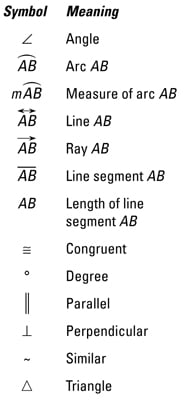##### Geometry For Dummies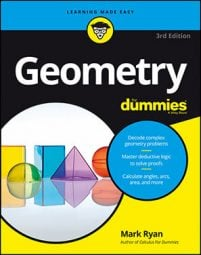Successfully understanding and studying geometry involves using strategies for your geometry proofs, knowing important equations, and being able to identify commonly used geometry symbols.

## Geometry formulas, theorems, properties, and more

What follows are over three dozen of the most important geometry formulas, theorems, properties, and so on that you use for calculations. If you get stumped while working on a geometry problem and can’t come up with a formula, this is the place to look.

### Circle formulas and theorems

• Circumference: C = 2πr or πd, where r is the radius of the circle and d is its diameter
• Area: AreaCircle = πr2
• Arc length: The length of an arc (part of the circumference) is equal to the circumference of the circle (2πr) times the fraction of the circle represented by the arc.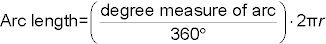• Sector area: The area of a sector (a pizza-slice shape cut out of a circle) is equal to the area of the circle (πr2) times the fraction of the circle represented by the sector.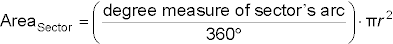• Measure of an angle . . .
• Chord-chord power theorem: When two chords of a circle intersect, the product of the parts of one chord is equal to the product of the parts of the other chord.
• Tangent-secant power theorem: When a tangent and a secant of a circle meet at an external point, the measure of the tangent squared is equal to the product of the secant’s external part and its total length.
• Secant-secant power theorem: When two secants of a circle meet at an external point, the product of one secant’s external part and its total length is equal to the product of the other secant’s external part and its total length.

### 3-D geometry calculations

• Flat-top objects (prisms and cylinders):
• Volume: VolFlat-top = areabase × height
• Surface area: SAFlat-top = 2 × areabase + arealateral rectangles
For a cylinder, the single lateral rectangle that wraps around the cylinder has a length equal to the circumference of the cylinder’s base; its width is the cylinder’s height.
• Pointy-top objects (pyramids and cones):
• Volume: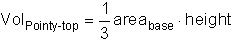• Surface area: SAPointy-top = areabase + arealateral triangles
For a pyramid, the base of each lateral triangle is a side of the pyramid’s base, and the height of the triangle is the slant height of the pyramid. For a cone, one “triangle” wraps around the cone; its base is equal to the circumference of the cone’s base, and its height is equal to the slant height of the cone.
• Sphere:

### Coordinate geometry

• Slope formula: Given two points (x1, y1) and (x2, y2), the slope of the line that goes through the points is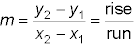It doesn’t matter which point is designated as (x1, y1) and which is designated as (x2, y2).

• The slopes of parallel lines are equal.
• The slopes of perpendicular lines are opposite reciprocals of each other.
• Midpoint formula: Given a segment with endpoints (x1, y1) and (x2, y2), the coordinates of its midpoint are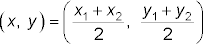It doesn’t matter which point is (x1, y1) and which is(x2, y2).
• Distance formula: Given two points (x1, y1) and (x2, y2), the distance between the points is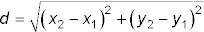It doesn’t matter which point is (x1, y1) and which is (x2, y2).
• Equations of a line:
• Slope-intercept form: y = mx + b, where m is the slope and b is the y-intercept
• Point-slope form: yy1 = m(xx1), where m is the slope and (x1, y1) is a point on the line
• Horizontal line: y = b, where b is the y-intercept
• Vertical line: x = a, where a is the x-intercept
• Equation of a circle: (xh)2 + (yk)2 = r2, where (h, k) is the center of the circle and r is its radius

## Proof strategies in geometry

Knowing how to write two-column geometry proofs provides a solid basis for working with theorems. Practicing these strategies will help you write geometry proofs easily in no time:

• Make a game plan. Try to figure out how to get from the givens to the prove conclusion with a plain English, commonsense argument before you worry about how to write the formal, two-column proof.

• Make up numbers for segments and angles. During the game plan stage, it’s sometimes helpful to make up arbitrary lengths for segments or measures for angles. Doing the math with those numbers (addition, subtraction, multiplication, or division) can help you understand how the proof works.

• Look for congruent triangles (and keep CPCTC in mind). In diagrams, try to find all pairs of congruent triangles. Proving one or more of these pairs of triangles congruent (with SSS, SAS, ASA, AAS, or HLR) will likely be an important part of the proof. Then you’ll almost certainly use CPCTC on the line right after you prove triangles congruent.

• Try to find isosceles triangles. Glance at the proof diagram and look for all isosceles triangles. If you find any, you’ll very likely use the if-sides-then-angles or the if-angles-then-sides theorem somewhere in the proof.

• Look for parallel lines. Look for parallel lines in the proof’s diagram or in the givens. If you find any, you’ll probably use one or more of the parallel-line theorems.

• Look for radii and draw more radii. Notice each and every radius of a circle and mark all radii congruent. Draw new radii to important points on the circle, but don’t draw a radius that goes to a point on the circle where nothing else is happening.

• Use all the givens. Geometry book authors don’t put irrelevant givens in proofs, so ask yourself why the author provided each given. Try putting each given down in the statement column and writing another statement that follows from that given, even if you don’t know how it’ll help you.

For each reason, check that

• All the ideas in the if clause appear in the statement column somewhere above the line youre checking.

• The single idea in the then clause also appears in the statement column on the same line.

You can also use this strategy to figure out what reason to use in the first place.

• Work backward. If you get stuck, jump to the end of the proof and work back toward the beginning. After looking at the prove conclusion, make a guess about the reason for that conclusion. Then use your if-then logic to figure out the second-to-last statement (and so on).

• Think like a computer. In a two-column proof, every single step in the chain of logic must be expressed, even if it’s the most obvious thing in the world. Doing a proof is like communicating with a computer: The computer won’t understand you unless every little thing is precisely spelled out.

• Do something. Before you give up on a proof, put whatever you understand down on paper. It’s quite remarkable how often putting something on paper triggers another idea, then another, and then another. Before you know it, you’ve finished the proof.

## Common geometry symbols

Using geometry symbols will save time and space when writing proofs, properties, and figuring formulas. Here are the most commonly used geometry symbols and their meanings: